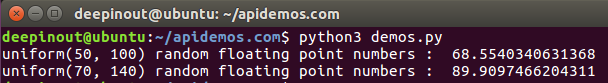# Python random uniform() 函数

## Python random uniform() 函数

Python random uniform() 方法将随机生成下一个实数，它在 [x,y] 范围内。

## Python random uniform() 语法

``````import random

random.uniform(x, y)``````

## Python random uniform() 参数

• x : 随机数的最小值，包含该值。
• y : 随机数的最大值，包含该值。

## Python random uniform() 实例

``````#!/usr/bin/python3
import random

print ("uniform(50, 100) random floating point numbers : ",  random.uniform(50, 100))
print ("uniform(70, 140) random floating point numbers : ",  random.uniform(70, 140))``````• 微信订阅
• 回顶